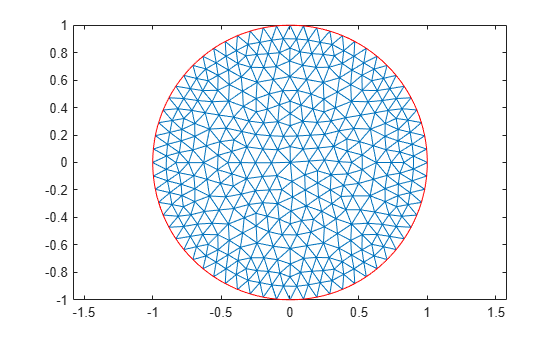Documentation

### This is machine translation

Mouseover text to see original. Click the button below to return to the English version of the page.

To view all translated materials including this page, select Country from the country navigator on the bottom of this page.

## Minimal Surface Problem on Unit Disk

This example shows how to solve a nonlinear elliptic problem.

### A Nonlinear PDE

A nonlinear problem is one whose coefficients not only depend on spatial coordinates, but also on the solution itself. An example of this is the minimal surface equation

``

on the unit disk, withon the boundary. To express this equation in toolbox form, note that the elliptic equation in toolbox syntax is

``

The PDE coefficient `c` is the multiplier of, namely

``is a function of the solution, so the problem is nonlinear. In toolbox syntax, you see that theandcoefficients are 0.

### Geometry

Create a PDE Model with a single dependent variable, and include the geometry of the unit disk. The `circleg` function represents this geometry. Plot the geometry and display the edge labels.

```numberOfPDE = 1; model = createpde(numberOfPDE); geometryFromEdges(model,@circleg); pdegplot(model,'EdgeLabels','on'); axis equal title 'Geometry with Edge Labels';```### Specify PDE Coefficients

```a = 0; f = 0; cCoef = @(region,state) 1./sqrt(1+state.ux.^2 + state.uy.^2); specifyCoefficients(model,'m',0,'d',0,'c',cCoef,'a',a,'f',f);```

### Boundary Conditions

Create a function that represents the boundary condition.

```bcMatrix = @(region,~)region.x.^2; applyBoundaryCondition(model,'dirichlet',... 'Edge',1:model.Geometry.NumEdges,... 'u',bcMatrix);```

### Generate Mesh

```generateMesh(model,'Hmax',0.1); figure; pdemesh(model); axis equal```### Solve PDE

Because the problem is nonlinear, solvepde invokes nonlinear solver. Observe the solver progress by setting the `SolverOptions.ReportStatistics` property of `model` to `'on'`.

```model.SolverOptions.ReportStatistics = 'on'; result = solvepde(model);```
```Iteration Residual Step size Jacobian: Full 0 1.8540e-02 1 2.8715e-04 1.0000000 2 1.2145e-06 1.0000000 ```
`u = result.NodalSolution;`

### Plot Solution

```figure; pdeplot(model,'XYData',u,'ZData',u); xlabel 'x' ylabel 'y' zlabel 'u(x,y)' title 'Minimal surface'```##### SupportGet trial now